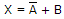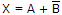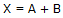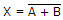# Digital Electronics - Logic Gates

### Exercise :: Logic Gates - General Questions

41.

What is the circuit number of the IC that contains four two-input AND gates in standard TTL?

 A. 7402 B. 7404 C. 7408 D. 7432

Explanation:

No answer description available for this question. Let us discuss.

42.

The terms "low speed" and "high speed," applied to logic circuits, refer to the ________.

 A. rise time B. fall time C. propagation delay time D. clock speed

Explanation:

No answer description available for this question. Let us discuss.

43.

The NOR logic gate is the same as the operation of the ________ gate with an inverter connected to the output.

 A. OR B. AND C. NAND D. none of the above

Explanation:

No answer description available for this question. Let us discuss.

44.

The logic expression for a NOR gate is ________.

 A.B.C.D.Explanation:

No answer description available for this question. Let us discuss.

45.

With regard to an AND gate, which statement is true?

 A. An AND gate has two inputs and one output. B. An AND gate has two or more inputs and two outputs. C. If one input to a 2-input AND gate is HIGH, the output reflects the other input. D. A 2-input AND gate has eight input possibilities.